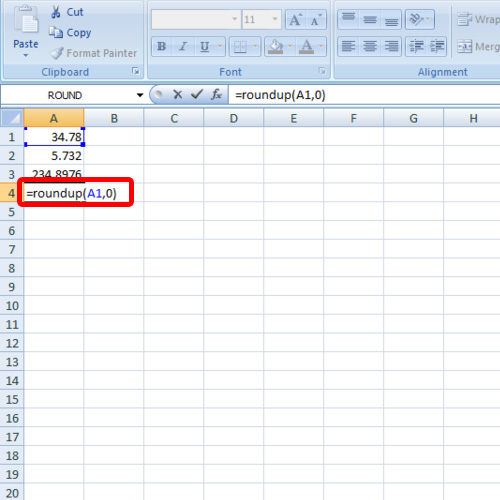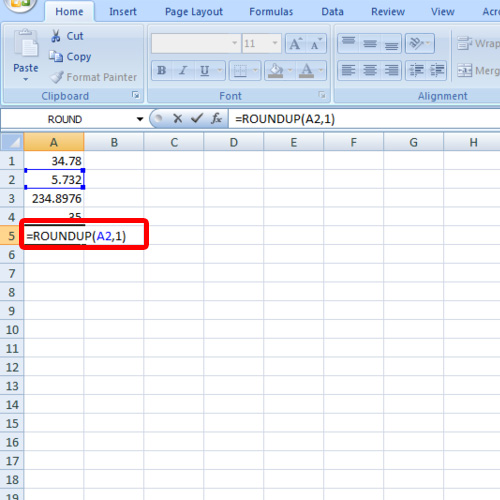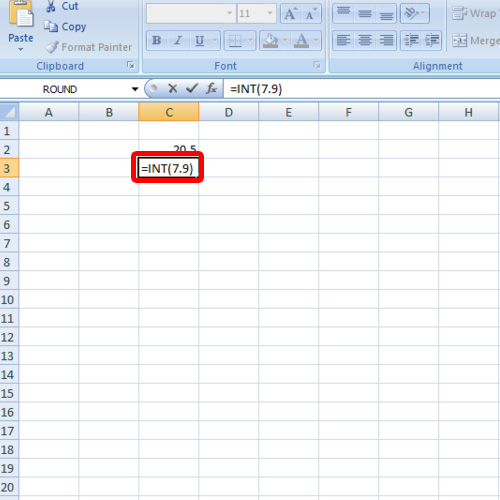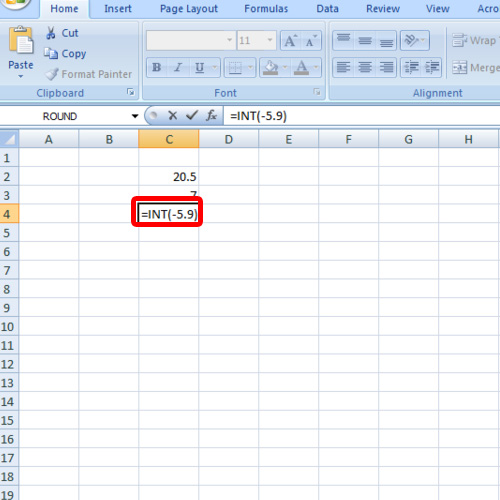## How to Round Number in Excel

In this tutorial, we will teach you how to round number in Excel. We will work with the roundup formula and the INT formula. The roundup formula can be used to round off the numbers while the INT formula converts the data in the cell to an integer and gets rid of the decimals.

Step 1 – Round off a number

Follow this step by step guide to learn how to round number in Excel.

First of all, open an Excel sheet. For the purpose of this tutorial, we will enter numbers with several decimal places in this sheet. Once you have entered the data you want to work with, go to the cell in which you want to place the roundup formula. Enter the following formula:

=roundup(A1,0)

In the formula, A1 contains the cell we want to round off while the zero in the formula means that we want to round it up to zero decimal places.Step 2 – Roundup formula

If you want to round cell A2 to one decimal place, insert the following formula:

=roundup(A2,1)

In this manner, you can use the roundup formula to round off numbers in Excel.Step 3 – Use INT function

Furthermore, we will teach you how to use the INT function. If you want to round off the number towards the lower end, type in

=INT(7.9)

7.9 will be rounded off to 7, the integer towards the lower end.Step 4 – Round off to the next integer

If you want to round off to the next number, simply insert the same formula just with a minus sign in front of the number.

For the purpose of this tutorial, we will apply the int function on 5.9. The minus sign will round off 5.9 into the next integer that will be 6.

In this manner, you can round off numbers in Excel.